FOR TEACHERS

Provide your students the opportunity to discover for themselves the wandering stars. Create a custom solar system and allow your students the chance to interrogate the heavens (SAMPLE). Will they postulate a heliocentric universe or discover the law of ellipses? By working with hypothetical solar system data, you can guarantee that students don't take the expedient route to "finishing" their assignments.

Adapt the planet viewer to teach any of a number of topics in observational astronomy.

FOR STUDENTS

If you've been assigned to observe your own private solar system, just type in the login given to you by your instructor, and click "login."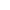FOR WEBMASTERS

Place today's sky on your webpage. Link here with one of these dynamic astronomy banners. Banner coding and instructions.

How to Calculate the Positions of the Planets: An Overview

This brief explanation describes the methods used in the simulation above to determine the Right Ascension (RA) and Declination (Dec) of the Sun, Mercury, Venus, Mars, Jupiter, and Saturn. It assumes some background in astronomy. However, there is a reasonably verbose glossary linked to what may be unfamiliar terms. In truth, this explanation is more for those wishing to know exactly what assumptions underlie the above simulation. However, this explanation does serve as a good case study in how to determine the planets' positions.

The simulation overlays the RA and Dec of the five planets visible to the naked eye and the sun atop an cylindrical projection of the Earth's sky. The name planet roughly translates to "wanderer." Knowing the geocentric model held for so long, many of my students simply assume the planets move about the sky in much the same manner as the moon or sun--steadily creeping eastward. They do not, and I developed this simulation to illustrate their true wandering nature.

Solving Kepler's Equation & Calculating Ephemerides

When first approaching this problem, I knew that I would need to solve Kepler's Equation and have a little fun with reference frames. I was surprised by the explanations available on the web. Most of them fell short of what I was looking for. They were either strictly qualitative or, if quantitative, unnecessarily opaque. This resulted mostly from having too few or poorly executed diagrams and illustrations. There were a few notable exceptions, and I have cited them in the references section. As I never formally studied celestial mechanics, these sources were my teachers and greatly helped acquaint me with the problem and its solutions. What I've attempted to do here is to weave together a relatively cogent "How to" on the solving of Kepler's Equation and the calculation of planetary positions in the Earth's sky.

Johannes Kepler (1571-1630) was a mathematician, astronomer, and Copernican. He believed that the Sun, not the Earth, lay at the center of the universe. He refined Copernicus's view of a heliocentric (Sun-centered) universe, making it into more than simply a competing theory for the geocentric (Earth-centered) model. Under Kepler it would become the superior predictive model. In his work Kepler formulated three laws of planetary motion first set down together in Harmonice Mundi (Harmonies of the World), 1618, and here they are.

Kepler's Laws:

1. The planets orbit about the Sun in elliptical orbits with the sun centered at one of the ellipse's two foci (Figure 1).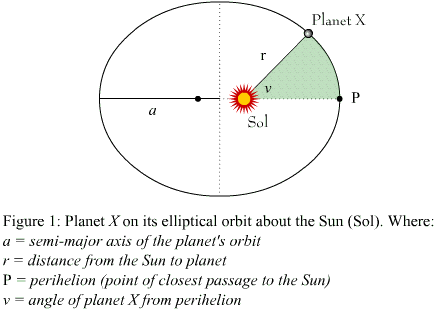2. An imaginary line connecting the sun and a planet sweeps out equal areas in equal times as the planet moves through its orbit. A consequence of this is that a planet moves fastest when closest to the Sun. Newton will have something to say about this.

3. The square of the period of a planet's orbit is proportional to its distance from the Sun cubed. When the units used for distance are Astronomical Units (AU) and time is measured in years, this relationship can be written explicitly as an equation relating the planet's period P and the semi-major axis of its orbit a (eq.1).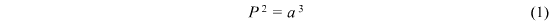Kepler's Laws meant that given only a handful of orbital parameters, one could say where a planet had been and would be. To state this explicitly, astronomers make use of Kepler's Equation (eq.2).Kepler's equation is a transcendental equation. This means there is no general solution. So to find the location of a planet at a time t, we must solve for that time using some numerical method. First let us work with what we have. NOTE: You may find it helpful to reference Figure 2 (pop-up) to help visualize some of the variables referenced here.

Only e is time independent. So we consult our orbital parameters for its value and then solve for the mean anomaly (eq.3), M in Kepler's Equation (eq.2). The mean anomaly is just the angle with the perihelion that the planet would have if the orbit was an ellipse with eccentricity = 0, i.e., a circle. We call the imaginary planet moving along such an orbit the mean planet. In such a case the planet would move with a velocity V = (2*PI)/Period .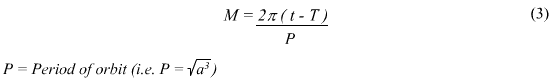As you can see, the mean anomaly is just the mean planet's velocity times the time elapsed since it was last at the perihelion.

We can now find the eccentric anomaly using some numerical method. This simulation makes use of successive approximation. Once we have a value for E with which we are happy, we can find the true anomaly (eq.4). The true anomaly is the ACTUAL angle between the perihelion and the planet.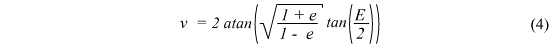From here it is a simple matter to find the planet's radial distance (eq.5) from the sun.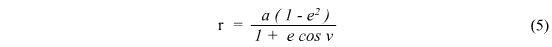We now have the planet's polar coordinates (r, v) within the plane of its orbit such that the X axis points from the Sun towards the Perihelion, point P.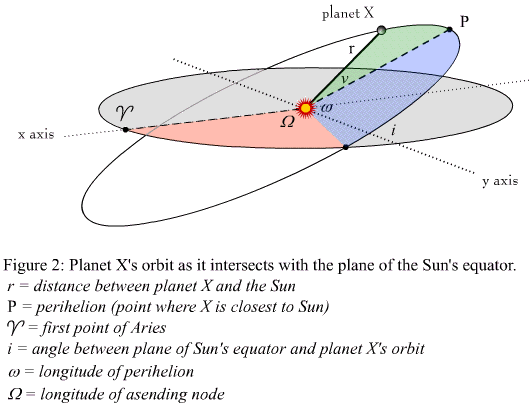Now we find the Heliocentric Ecliptic coordinates (x, y, z) for the planet by converting from polar to cartesian coordinates and rotating the frame such that the X axis points towards the first point of Aries.We then rotate the coordinates into Heliocentric Equatorial coordinates (X, Y, Z), making use the matrix below.However, our display shows the positions of the planets from the Earth. So we need to switch our vantage point to that of a geocentric system. To do this we first repeat the above process, solving for the Earth's Heliocentric Equatorial coordinates. We want to know the Sun's Geocentric coordinates. So here we will approximate this as the inverse of Earth's heliocentric coordinates. This is the same method used to find the Sun's location for display. It is important to note, however, that this is just an approximation, as what we really find is not the location of the Earth but rather that of the Earth-Moon system's barycenter. This simplification is responsible for limiting the simulation's accuracy. Note: This is not an issue for the Build Your Own Solar System simulation for teachers as the hypothetical "Earth" has no moon in that simulation. We then add the Sun's geocentric coordinates to those of the heliocentric coordinates of our planet. This shifts the coordinates, giving us the Geocentric equatorial coordinates (xp, yp, zp) for the planet.Having the planet's Geocentric coordinates, it is a simple matter to convert them into Right Ascension and Declination. Note: Watch you signs here; if you're not careful, it WILL get messy.That's it. We can now solve for many discreet times and collect the data into tables to construct ephemerides. If you are interested in finding more accurate calculations for the planets' positions, consider buying a copy of the Astronomical Almanac from the US Naval Observatory or making use of JPL's Horizons system.

• Astronomical Almanac (ordering info)
• JPL's Horizons system

1. To anyone interested in why it is the orbits of the planets are elliptical, I suggest finding a copy of D. & J. Goodstein's Feynman's Lost Lecture: The Motion of Planets Around the Sun. W. W. Norton & Company. New York, NY. 1996.

2. A copy of Kepler's Harmonice Mundi (Harmonies of the World) as well as many other ground breaking texts in astronomy have been compiled into one tome: Stephen Hawking's On The Shoulders of Giants: The Great Works of Physics and Astronomy.

3. For what I found to be the most rigorous on-line handling of this material, try Dr. J. B. Tatum's Celestial Mechanics: http://orca.phys.uvic.ca/~tatum/celmechs.html (Link current as of April 2004).

4. The orbital parameters used here came from the JPL Solar System Dynamics Group's "Planetary Orbital Elements," JPL Solar System Dynamics: http://ssd.jpl.nasa.gov/elem_planets.html. (Link current as of April 2004).

Glossary of Terms

Ascending node: The point of intersection between a planet's orbit and the plane of the Sun's equator, where the planet is moving northward ("upward") across the plane of the Sun's equator.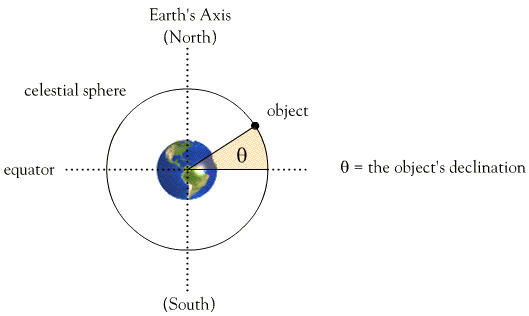Descending node: The point of intersection between a planet's orbit and the plane of the Sun's equator, where the planet is moving southward ("downward") across the plane of the Sun's equator.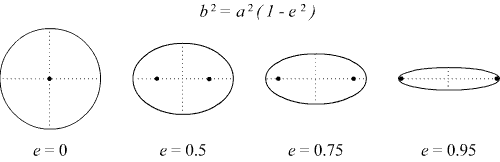Above are four ellipses with varying eccentricities. The first is a circle.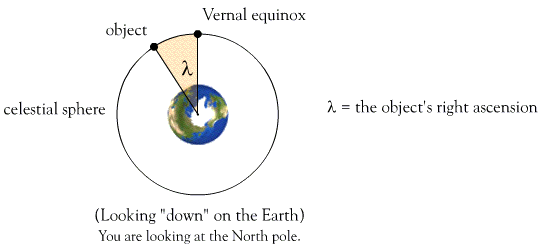Vector: A quantity consisting of both direction and magnitude (e.g., velocity).

• ← Do you like flowcharts? →